# Drawing with Cairo II

last modified July 16, 2020

In this part of the PyGTK programming tutorial, we will continue drawing with the Cairo library.

## Donut

In the following example we create a complex shape by rotating a bunch of ellipses.

donut.py
```#!/usr/bin/python

# ZetCode PyGTK tutorial
#
# This program creates a donut
# with cairo library
#
# author: jan bodnar
# website: zetcode.com
# last edited: February 2009

import gtk
import math

class PyApp(gtk.Window):

def __init__(self):
super(PyApp, self).__init__()

self.set_title("Donut")
self.set_size_request(350, 250)
self.set_position(gtk.WIN_POS_CENTER)

self.connect("destroy", gtk.main_quit)

darea = gtk.DrawingArea()
darea.connect("expose-event", self.expose)
self.add(darea)

self.show_all()

def expose(self, widget, event):

cr = widget.window.cairo_create()

cr.set_line_width(0.5)

w = self.allocation.width
h = self.allocation.height

cr.translate(w/2, h/2)
cr.arc(0, 0, 120, 0, 2*math.pi)
cr.stroke()

for i in range(36):
cr.save()
cr.rotate(i*math.pi/36)
cr.scale(0.3, 1)
cr.arc(0, 0, 120, 0, 2*math.pi)
cr.restore()
cr.stroke()

PyApp()
gtk.main()
```

In this example, we create a donut. The shape resembles a cookie, hence the name donut.

```cr.translate(w/2, h/2)
cr.arc(0, 0, 120, 0, 2*math.pi)
cr.stroke()
```

In the beginning there is an ellipse.

```for i in range(36):
cr.save()
cr.rotate(i*math.pi/36)
cr.scale(0.3, 1)
cr.arc(0, 0, 120, 0, 2*math.pi)
cr.restore()
cr.stroke()
```

After several rotations, there is a donut. We insulate each rotate and scale operations from one another with the `save()` and `restore()` methods.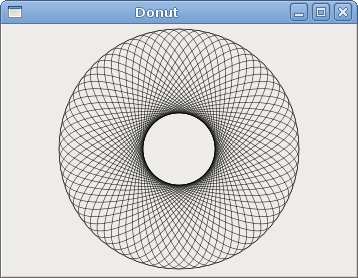Figure: Donut

## Gradients

In computer graphics, gradient is a smooth blending of shades from light to dark or from one colour to another. In 2D drawing programs and paint programs, gradients are used to create colourful backgrounds and special effects as well as to simulate lights and shadows. (answers.com)

gradients.py
```#!/usr/bin/python

# ZetCode PyGTK tutorial
#
# This program works with
# gradients in cairo
#
# author: jan bodnar
# website: zetcode.com
# last edited: February 2009

import gtk
import cairo

class PyApp(gtk.Window):

def __init__(self):
super(PyApp, self).__init__()

self.set_title("Gradients")
self.set_size_request(340, 390)
self.set_position(gtk.WIN_POS_CENTER)

self.connect("destroy", gtk.main_quit)

darea = gtk.DrawingArea()
darea.connect("expose-event", self.expose)
self.add(darea)

self.show_all()

def expose(self, widget, event):

cr = widget.window.cairo_create()
lg1 = cairo.LinearGradient(0.0, 0.0, 350.0, 350.0)

count = 1

i = 0.1
while i < 1.0:
if count % 2:
lg1.add_color_stop_rgba(i, 0, 0, 0, 1)
else:
lg1.add_color_stop_rgba(i, 1, 0, 0, 1)
i = i + 0.1
count = count + 1

cr.rectangle(20, 20, 300, 100)
cr.set_source(lg1)
cr.fill()

lg2 = cairo.LinearGradient(0.0, 0.0, 350.0, 0)

count = 1

i = 0.05
while i < 0.95:
if count % 2:
lg2.add_color_stop_rgba(i, 0, 0, 0, 1)
else:
lg2.add_color_stop_rgba(i, 0, 0, 1, 1)
i = i + 0.025
count = count + 1

cr.rectangle(20, 140, 300, 100)
cr.set_source(lg2)
cr.fill()

lg3 = cairo.LinearGradient(20.0, 260.0,  20.0, 360.0)
lg3.add_color_stop_rgba(0.1, 0, 0, 0, 1)
lg3.add_color_stop_rgba(0.5, 1, 1, 0, 1)
lg3.add_color_stop_rgba(0.9, 0, 0, 0, 1)

cr.rectangle(20, 260, 300, 100)
cr.set_source(lg3)
cr.fill()

PyApp()
gtk.main()
```

In our example, we draw three rectangles with three different gradients.

```lg1 = cairo.LinearGradient(0.0, 0.0, 350.0, 350.0)
```

Here we create a linear gradient pattern. The parameters specify the line, along which we draw the gradient. In our case it is a vertical line.

```lg3 = cairo.LinearGradient(20.0, 260.0,  20.0, 360.0)
lg3.add_color_stop_rgba(0.1, 0, 0, 0, 1)
lg3.add_color_stop_rgba(0.5, 1, 1, 0, 1)
lg3.add_color_stop_rgba(0.9, 0, 0, 0, 1)
```

We define colour stops to produce our gradient pattern. In this case, the gradient is a blending of black and yellow colours. By adding two black and one yellow stops, we create a horizontal gradient pattern. What do these stops actually mean? In our case, we begin with black colour, which will stop at 1/10 of the size. Then we begin to gradually paint in yellow, which will culminate at the centre of the shape. The yellow colour stops at 9/10 of the size, where we begin painting in black again, until the end.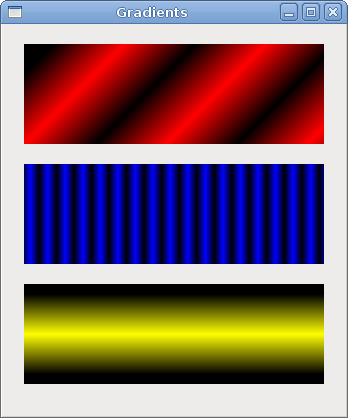Figure: Gradients

## Puff

In the following example, we create a puff effect. The example will display a growing centered text that will gradually fade out from some point. This is a very common effect, which you can often see in flash animations.

puff.py
```#!/usr/bin/python

# ZetCode PyGTK tutorial
#
# This program creates a puff
# effect
#
# author: jan bodnar
# website: zetcode.com
# last edited: February 2009

import gtk
import glib
import cairo

class PyApp(gtk.Window):

def __init__(self):
super(PyApp, self).__init__()

self.set_title("Puff")
self.resize(350, 200)
self.set_position(gtk.WIN_POS_CENTER)

self.connect("destroy", gtk.main_quit)

self.darea = gtk.DrawingArea()
self.darea.connect("expose-event", self.expose)
self.add(self.darea)

self.timer = True
self.alpha = 1.0
self.size = 1.0

glib.timeout_add(14, self.on_timer)

self.show_all()

def on_timer(self):
if not self.timer: return False

self.darea.queue_draw()
return True

def expose(self, widget, event):

cr = widget.window.cairo_create()

w = self.allocation.width
h = self.allocation.height

cr.set_source_rgb(0.5, 0, 0)
cr.paint()

cr.select_font_face("Courier", cairo.FONT_SLANT_NORMAL, cairo.FONT_WEIGHT_BOLD)

self.size = self.size + 0.8

if self.size > 20:
self.alpha = self.alpha - 0.01

cr.set_font_size(self.size)
cr.set_source_rgb(1, 1, 1)

(x, y, width, height, dx, dy) = cr.text_extents("ZetCode")

cr.move_to(w/2 - width/2, h/2)
cr.text_path("ZetCode")
cr.clip()
cr.stroke()
cr.paint_with_alpha(self.alpha)

if self.alpha <= 0:
self.timer = False

PyApp()
gtk.main()
```

The example creates a growing and fading text on the window.

```glib.timeout_add(14, self.on_timer)
```

Every 14 ms the `on_timer()` method is called.

```def on_timer(self):
if not self.timer: return False

self.darea.queue_draw()
return True
```

In the `on_timer()` method, we call the `queue_draw()` method upon the drawing area, which triggers the expose signal.

```cr.set_source_rgb(0.5, 0, 0)
cr.paint()
```

We set the background colour to dark red colour.

```self.size = self.size + 0.8
```

Each cycle, the font size will grow by 0.8 units.

```if self.size > 20:
self.alpha = self.alpha - 0.01
```

The fading out begins after the font size is bigger than 20.

```(x, y, width, height, dx, dy) = cr.text_extents("ZetCode")
```

We get the text metrics.

```cr.move_to(w/2 - width/2, h/2)
```

We use the text metrics to center the text on the window.

```cr.text_path("ZetCode")
cr.clip()
```

We get the path of the text and set the current clip region to it.

```cr.stroke()
cr.paint_with_alpha(self.alpha)
```

We paint the current path and take alpha value into account.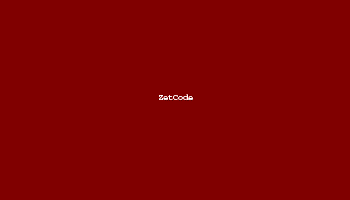Figure: Puff

## Reflection

In the next example we show a reflected image. This beautiful effect makes an illusion as if the image was reflected in water.

reflection.py
```#!/usr/bin/python
# -*- coding: utf-8 -*-

# ZetCode PyGTK tutorial
#
# This program creates an
# image reflection
#
# author: Jan Bodnar
# website: zetcode.com
# last edited: April 2011

import gtk
import cairo
import sys

class PyApp(gtk.Window):

def __init__(self):
super(PyApp, self).__init__()

self.set_title("Reflection")
self.resize(300, 350)
self.set_position(gtk.WIN_POS_CENTER)

self.connect("destroy", gtk.main_quit)

darea = gtk.DrawingArea()
darea.connect("expose-event", self.expose)
self.add(darea)

try:
self.surface = cairo.ImageSurface.create_from_png("slanec.png")
except Exception, e:
print e.message
sys.exit(1)

self.imageWidth = self.surface.get_width()
self.imageHeight = self.surface.get_height()
self.gap = 40
self.border = 20

self.show_all()

def expose(self, widget, event):

cr = widget.window.cairo_create()

w = self.allocation.width
h = self.allocation.height

lg = cairo.LinearGradient(w/2, 0, w/2, h*3)
lg.add_color_stop_rgba(0, 0, 0, 0, 1)
lg.add_color_stop_rgba(h, 0.2, 0.2, 0.2, 1)

cr.set_source(lg)
cr.paint()

cr.set_source_surface(self.surface, self.border, self.border)
cr.paint()

alpha = 0.7
step = 1.0 / self.imageHeight

cr.translate(0, 2 * self.imageHeight + self.gap)
cr.scale(1, -1)

i = 0

while(i < self.imageHeight):

cr.rectangle(self.border, self.imageHeight-i, self.imageWidth, 1)

i = i + 1

cr.save()
cr.clip()
cr.set_source_surface(self.surface, self.border, self.border)
alpha = alpha - step
cr.paint_with_alpha(alpha)
cr.restore()

PyApp()
gtk.main()
```

The example shows a reflected castle.

```lg = cairo.LinearGradient(w/2, 0, w/2, h*3)
lg.add_color_stop_rgba(0, 0, 0, 0, 1)
lg.add_color_stop_rgba(h, 0.2, 0.2, 0.2, 1)

cr.set_source(lg)
cr.paint()
```

The background is filled with a gradiet paint. The paint is a smooth blending from black to dark gray.

```cr.translate(0, 2 * self.imageHeight + self.gap)
cr.scale(1, -1)
```

This code flips the image and translates it below the original image. The translation operation is necessary, because the scaling operation makes the image upside down and translates the image up. To understand what happens, simply take a photograph and place it on the table. Now flip it.

```cr.rectangle(self.border, self.imageHeight-i, self.imageWidth, 1)

i = i + 1

cr.save()
cr.clip()
cr.set_source_surface(self.surface, self.border, self.border)
alpha = alpha - step
cr.paint_with_alpha(alpha)
cr.restore()
```

This is the final part. We make the second image transparent. But the transparency is not constant. The image gradually fades out. The reflected image is draw line by line. The `clip()` method restricts the drawing to the rectangle of height 1. The `paint_with_alpha()` takes the transparency into account when painting the current clip of the image surface.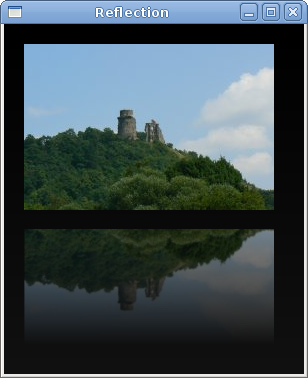Figure: Reflection

## Waiting

In this examle, we use transparency effect to create a waiting demo. We will draw 8 lines that will gradually fade out creating an illusion that a line is moving. Such effects are often used to inform users, that a lengthy task is going on behind the scenes. An example is streaming video over the internet.

waiting.py
```#!/usr/bin/python

# ZetCode PyGTK tutorial
#
# This program creates an
# waiting effect
#
# author: jan bodnar
# website: zetcode.com
# last edited: February 2009

import gtk
import glib
import math
import cairo

trs = (
( 0.0, 0.15, 0.30, 0.5, 0.65, 0.80, 0.9, 1.0 ),
( 1.0, 0.0,  0.15, 0.30, 0.5, 0.65, 0.8, 0.9 ),
( 0.9, 1.0,  0.0,  0.15, 0.3, 0.5, 0.65, 0.8 ),
( 0.8, 0.9,  1.0,  0.0,  0.15, 0.3, 0.5, 0.65 ),
( 0.65, 0.8, 0.9,  1.0,  0.0,  0.15, 0.3, 0.5 ),
( 0.5, 0.65, 0.8, 0.9, 1.0,  0.0,  0.15, 0.3 ),
( 0.3, 0.5, 0.65, 0.8, 0.9, 1.0,  0.0,  0.15 ),
( 0.15, 0.3, 0.5, 0.65, 0.8, 0.9, 1.0,  0.0, )
)

class PyApp(gtk.Window):

def __init__(self):
super(PyApp, self).__init__()

self.set_title("Waiting")
self.set_size_request(250, 150)
self.set_position(gtk.WIN_POS_CENTER)

self.connect("destroy", gtk.main_quit)

self.darea = gtk.DrawingArea()
self.darea.connect("expose-event", self.expose)
self.add(self.darea)

self.count = 0

glib.timeout_add(100, self.on_timer)

self.show_all()

def on_timer(self):
self.count = self.count + 1
self.darea.queue_draw()
return True

def expose(self, widget, event):

cr = widget.window.cairo_create()

cr.set_line_width(3)
cr.set_line_cap(cairo.LINE_CAP_ROUND)

w = self.allocation.width
h = self.allocation.height

cr.translate(w/2, h/2)

for i in range(8):
cr.set_source_rgba(0, 0, 0, trs[self.count%8][i])
cr.move_to(0.0, -10.0)
cr.line_to(0.0, -40.0)
cr.rotate(math.pi/4)
cr.stroke()

PyApp()
gtk.main()
```

We draw eight lines with eight different alpha values.

```glib.timeout_add(100, self.on_timer)
```

We use a timer function to create animation.

```trs = (
( 0.0, 0.15, 0.30, 0.5, 0.65, 0.80, 0.9, 1.0 ),
...
)
```

This is a two dimensional tuple of transparency values used in this demo. There are 8 rows, each for one state. Each of the 8 lines will continuously use these values.

```cr.set_line_width(3)
cr.set_line_cap(cairo.LINE_CAP_ROUND)
```

We make the lines a bit thicker, so that they are better visible. We draw the lines with rounded caps. They look then better.

```cr.set_source_rgba(0, 0, 0, trs[self.count%8][i]
```

Here we define the transparency value for a line.

```cr.move_to(0.0, -10.0)
cr.line_to(0.0, -40.0)
cr.rotate(math.pi/4)
cr.stroke()
```

These code lines will draw each of the eight lines.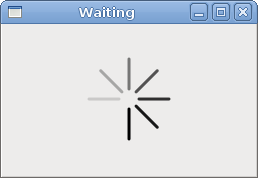Figure: Waiting

In this chapter of the PyGTK programming library, we did some more advanced drawing with the Cairo library.